Cyber Monday Sale: ALL MCAT Products & Admissions Services

MCAT Content / Gas Phase / Kinetic Molecular Theory Of Gases

### Kinetic Molecular Theory of Gases

Topic: Gas Phase

According to Kinetic Molecular Theory, an increase in temperature will increase the average kinetic energy of the molecules.

The Kinetic Molecular Theory of Gases comes from observations that scientists made about gases to explain their macroscopic properties. The following are the basic assumptions of the Kinetic Molecular Theory:

1. The volume occupied by the individual particles of a gas is negligible compared to the volume of the gas itself.
2. The particles of an ideal gas exert no attractive forces on each other or on their surroundings.
3. Gas particles are in a constant state of random motion and move in straight lines until they collide with another body.
4. The collisions exhibited by gas particles are completely elastic; when two molecules collide, total kinetic energy is conserved.
5. The average kinetic energy of gas molecules is directly proportional to absolute temperature only; this implies that all molecular motion ceases if the temperature is reduced to absolute zero.

Charles’ Law states that at constant pressure, the volume of a gas increases or decreases by the same factor as its temperature. This can be written as: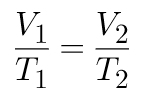According to Kinetic Molecular Theory, an increase in temperature will increase the average kinetic energy of the molecules. As the particles move faster, they will likely hit the edge of the container more often. If the reaction is kept at constant pressure, they must stay farther apart, and an increase in volume will compensate for the increase in particle collision with the surface of the container.

Boyle’s Law states that at constant temperature, the absolute pressure and volume of a given mass of confined gas are inversely proportional. This relationship is shown by the following equation: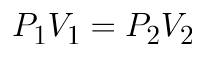At a given temperature, the pressure of a container is determined by the number of times gas molecules strike the container walls. If the gas is compressed to a smaller volume, then the same number of molecules will strike against a smaller surface area; the number of collisions against the container will increase, and, by extension, the pressure will increase as well. Increasing the kinetic energy of the particles will increase the pressure of the gas.

Heat capacity (usually denoted by a capital C, often with subscripts), or thermal capacity, is the measurable physical quantity that characterizes the amount of heat required to change a substance’s temperature by a given amount. An object’s heat capacity (symbol C) is defined as the ratio of the amount of heat energy transferred to an object to the resulting increase in temperature of the object.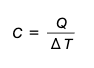The heat capacity of most systems is not a constant. Rather, it depends on the state variables of the thermodynamic system under study. In particular, it is dependent on temperature itself, as well as on the pressure and the volume of the system, and the ways in which pressures and volumes have been allowed to change while the system has passed from one temperature to another. Different measurements of heat capacity can therefore be performed, most commonly at constant pressure and constant volume. The values thus measured are usually subscripted (by p and V, respectively) to indicate the definition. Gases and liquids are typically also measured at constant volume. An ideal gas has different specific heat capacities under constant volume or constant pressure conditions.

The heat capacity at constant volume of nR = 1 J·K−1 of any gas, including an ideal gas is: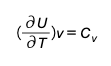This represents the dimensionless heat capacity at constant volume; it is generally a function of temperature due to intermolecular forces. For moderate temperatures, the constant for a monoatomic gas is cv=3/2 while for a diatomic gas it is cv=5/2 (see ). Macroscopic measurements on heat capacity provide information on the microscopic structure of the molecules.

The heat capacity at constant pressure of 1 J·K−1 ideal gas is: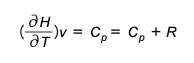where H=U+pV is the enthalpy of the gas.

Boltzmann’s constant (kB or k) is a physical constant that relates the average relative kinetic energy of particles in a gas with the temperature of the gas. The Boltzmann constant is defined to be exactly 1.380649×10−23 J/K.

Practice Questions

Heat engines and work

Ideal gases in medicine

MCAT Official Prep (AAMC)

Key Points

• Kinetic Molecular Theory states that gas particles are in constant motion and exhibit perfectly elastic collisions.

• Kinetic Molecular Theory can be used to explain both Charles’ and Boyle’s Laws.

• The average kinetic energy of a collection of gas particles is directly proportional to absolute temperature only.

• The Boltzmann constant is defined to be exactly 1.380649×10−23 J/K.

• The specific heat at constant volume for a gas is given as• The specific heat at constant pressure for an ideal gas is given as• The heat capacity ratio (or adiabatic index) is the ratio of the heat capacity at constant pressure to heat capacity at constant volume.

Key Terms

Kinetic energy: The energy possessed by an object because of its motion.

Ideal gas: A gas whose particles exhibit no attractive interactions.

Heat capacity: The amount of heat to raise the temperature of 1 mole by 1 K; Q = mCΔT.

Boltzmann constant: Relates kinetic energy and absolute temperature of a gas; k = 1.3807 x 10^-23 (J/K ).

adiabatic index: The ratio of the heat capacity at constant pressure to heat capacity at constant volume.

specific heat: The ratio of the amount of heat needed to raise the temperature of a unit mass of substance by a unit degree to the amount of heat needed to raise that of the same mass of water by the same amount.

Billing Information